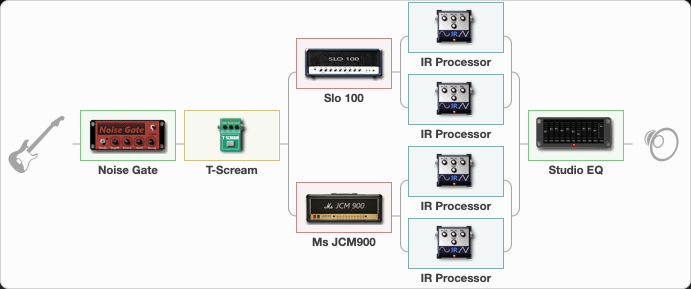Discussion in 'ToneLib-GFX presets' started by grigoriy0192873, Mar 20, 2023.

1. ### grigoriy0192873Active Member

Preset name: Cryptic Writings

Effects chain:Effect: "Noise Gate" (Dynamics / Filter), active - "yes"
{
"Mode" = Auto
"Depth" = 50
"Threshold" = 50
"Attack" = 0
"Hold" = 5
"Decay" = 635
}

Effect: "T-Scream" (Overdrive / Distortion), active - "yes"
{
"Drive" = 0
"Tone" = 80
"Level" = 100
}

Effect: "Splitter" (Dynamics / Filter)
{
"A-Bypass" = Off
"A-Pan" = -100
"A-Level" = 55
"B-Bypass" = Off
"B-Pan" = 100
"B-Level" = 55
"Width" = 100

'A' branch:
{

Effect: "Slo 100" (Amp simulators), active - "yes"
{
"Gain" = 30
"Bass" = 30
"Middle" = 50
"Treble" = 50
"Presence" = 50
"Master" = 50
"Level (dB)" = 8
}

Effect: "Splitter" (Dynamics / Filter)
{
"A-Bypass" = Off
"A-Pan" = -25
"A-Level" = 55
"B-Bypass" = Off
"B-Pan" = 25
"B-Level" = 55
"Width" = 0

'A' branch:
{

Effect: "IR Processor" (Cabinets), active - "yes"
{
"IR" = Ride_IR
"Low Cut (Hz)" = 0
"Hi Cut (kHz)" = 20.0
"Mix" = 100
"Level (dB)" = 0
}
}
'B' branch:
{

Effect: "IR Processor" (Cabinets), active - "yes"
{
"Low Cut (Hz)" = 0
"Hi Cut (kHz)" = 20.0
"Mix" = 100
"Level (dB)" = 0
}
}
}
}
'B' branch:
{

Effect: "Ms JCM900" (Amp simulators), active - "yes"
{
"Gain" = 50
"Bass" = 30
"Middle" = 70
"Treble" = 80
"Presence" = 50
"Master" = 50
"Level (dB)" = 6
}

Effect: "Splitter" (Dynamics / Filter)
{
"A-Bypass" = Off
"A-Pan" = -25
"A-Level" = 55
"B-Bypass" = Off
"B-Pan" = 25
"B-Level" = 55
"Width" = 0

'A' branch:
{

Effect: "IR Processor" (Cabinets), active - "yes"
{
"IR" = Ride_IR
"Low Cut (Hz)" = 0
"Hi Cut (kHz)" = 20.0
"Mix" = 100
"Level (dB)" = 0
}
}
'B' branch:
{

Effect: "IR Processor" (Cabinets), active - "yes"
{
"Low Cut (Hz)" = 0
"Hi Cut (kHz)" = 20.0
"Mix" = 100
"Level (dB)" = 0
}
}
}
}
}

Effect: "Studio EQ" (Dynamics / Filter), active - "yes"
{
"31 Hz" = 0
"62 Hz" = 0
"125 Hz" = 5
"250 Hz" = -1
"500 Hz" = -3
"1 kHz" = 6
"2 kHz" = -3
"4 kHz" = 3
"8 kHz" = 0
"16 kHz" = 15
"Level (dB)" = -5
}

Note: You will need to download and install the ToneLib-GFX software to use the preset.